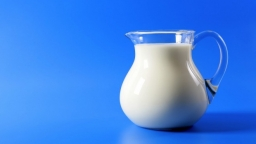# In the dairy

There were three times more milk packages in the dairy than half a liter. Four times more liters remained when sold in 10 liters and ten half-liter containers than in half-liter packages. How many packages were there originally?

a =  210
b =  70

### Step-by-step explanation:

a=3b
a-10 = 4·(b-20)

a=3·b
a-10 = 4·(b-20)

a-3b = 0
a-4b = -70

Row 2 - Row 1 → Row 2
a-3b = 0
-b = -70

b = -70/-1 = 70
a = 0+3b = 0+3 · 70 = 210

a = 210
b = 70

Our linear equations calculator calculates it.Did you find an error or inaccuracy? Feel free to write us. Thank you!

Tips for related online calculators
Do you have a linear equation or system of equations and looking for its solution? Or do you have a quadratic equation?